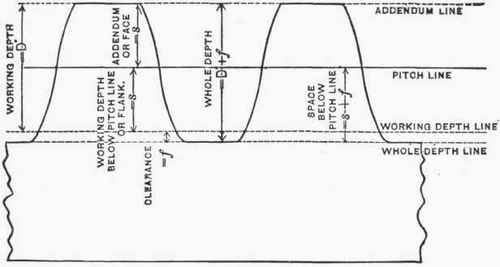There are certain terms relating to gears with which the mechanic should be familiar. Some of the most important of these are explained below. (See Fig. 155.)

Spur. - Spur originally meant a projection or tooth, but is now used to distinguish spur gears from other varieties of gears, such as bevel gears and worm gears.

Pitch Circle. - The pitch circle of a gear is the distance around the teeth and is the same size as the friction rollers or cylinders would be if no teeth were present: i.e., when two spur gears roll together their pitch circles are considered to be constantly in contact.

Pitch Diameter. - The pitch diameter of a gear is the diameter of the pitch circle.Fig. 154. - Train of Gears.

Circular Pitch. - The circular pitch of a gear is the distance measured along the pitch circle from the center line of one tooth to the center line of the next.Fig. 155.

Diametral Pitch. - The diametral pitch of a gear is the number of teeth per inch of pitch diameter. (For example, if a gear has 30 teeth and its pitch diameter is 3 in., the diametral pitch is 30 / 3 or 10.)

Addendum. - The addendum of a gear is the height of the top part of the tooth, i.e., the distance from the pitch line to the point of the tooth.

Dedendum. - The dedendum of a gear is the working depth of the tooth below the pitch line. It is always equal to the addendum.

Working Depth. - The working depth of the teeth of a following gear is the depth to which the teeth in the meshing gear center into the spaces between the teeth of the first or driving gear.

Clearance. - The clearance of a gear is the amount that the tooth space is cut deeper than the working depth. (The working depth of a tooth equals the sum of the addendum and the dedendum, while its total depth equals the sum of the addendum, dedendum, and clearance.)